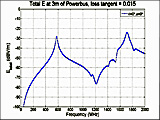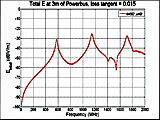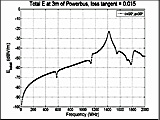﻿ Modeling Powerbus with EMAP

Modeling a Powerbus with EMAP

 Geometry and setup Double-sided PCB: Size: 125 mm × 100 mm × 1 mm Top and bottom metal: PEC Dielectric: FR4, εr = 4.5, dielectric loss tangent = 0.015 Excitation: Current source (0.02 A, 50 ohms) Mesh Setup: Dielectric mesh size: 1 mm × 8 mm PEC mesh size: 8 mm × 8 mm Frequency: 5 MHz - 2 GHz, Step Size = 5 MHz Simulation result Simulation Time: 22 hrs Number of triangles: 952 Number of tetrahedra: 2390 Number of edges: 3560 Decisions the user must make that affect the accuracy of the result Thickness of dielectric: 1 mm Number of triangles and tetrahedra: We used a commercial mesh generator that created 952 triangles and 2390 tetrahedra. Aspect ratio of triangles and tetrahedra: less than 5 Comments How is a powerbus modeled in EMAP? In EMAP the powerbus is modeled as a slab of dielectric with two PEC sheets attached to the top and bottom surfaces of the slab. The relative permittivity and the loss tangent of the dielectric are assigned in the input file. What kind of source can be used for a powerbus in EMAP and how is it placed? For the Powerbus model, a current source can be applied in the dielectric on one inner edge connecting the two metal sheets. A resistive load can be assigned to the same edge of the source and serves as the source resistance. What kind of mesh is used for the powerbus in EMAP? In EMAP, all the external or surface metal is meshed as triangles. The density and aspect ratio can be controlled with the meshing software. The dielectric part is meshed as tetrahedra. At the interface where the surface metal joins the faces of the tetrahedra, the surface metal triangles exactly overlap with the tetrahedra faces. This was accomplished using commercial meshing software. What simulation methods are used for the powerbus in EMAP? This model has metal, dielectrics, and calculates far-field radiation. The Method of Moments (MOM) part of the code is used for the metal triangles; and the Finite Element Method (FEM) part is used for the dielectric. The EMAP code couples these parts at the interface. In this case, the interface was defined to be the surface of the dielectric slab.
 Screen shotsFig. 4. Electric field at 3 m, θ=0°, φ=0°Fig. 5. Electric field at 3 m, θ=90°, φ=0°Fig. 6. Electric field at 3 m, θ=90°, φ=90°